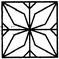Courses

# Pattern Completion Quiz III, Non Verbal Reasoning

## 25 Questions MCQ Test Non Verbal Reasoning | Pattern Completion Quiz III, Non Verbal Reasoning

Description
This mock test of Pattern Completion Quiz III, Non Verbal Reasoning for LR helps you for every LR entrance exam. This contains 25 Multiple Choice Questions for LR Pattern Completion Quiz III, Non Verbal Reasoning (mcq) to study with solutions a complete question bank. The solved questions answers in this Pattern Completion Quiz III, Non Verbal Reasoning quiz give you a good mix of easy questions and tough questions. LR students definitely take this Pattern Completion Quiz III, Non Verbal Reasoning exercise for a better result in the exam. You can find other Pattern Completion Quiz III, Non Verbal Reasoning extra questions, long questions & short questions for LR on EduRev as well by searching above.
QUESTION: 1

### Directions to Solve In each of the following questions, select a figure from amongst the four alternatives, which when placed in the blank space of figure (X) would complete the pattern. Question - Identify the figure that completes the pattern.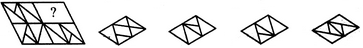(X)                 (1)             (2)              (3)             (4)

Solution: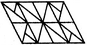QUESTION: 2

### Directions to Solve In each of the following questions, select a figure from amongst the four alternatives, which when placed in the blank space of figure (X) would complete the pattern. Question - Identify the figure that completes the pattern.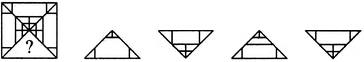(X)                 (1)             (2)              (3)             (4)

Solution: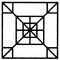QUESTION: 3

### Directions to Solve In each of the following questions, select a figure from amongst the four alternatives, which when placed in the blank space of figure (X) would complete the pattern. Question - Identify the figure that completes the pattern.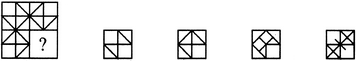(X)                 (1)             (2)              (3)             (4)

Solution: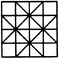QUESTION: 4

In each of the following questions, select a figure from amongst the four alternatives, which when placed in the blank space of figure (X) would complete the pattern.

Question -

Identify the figure that completes the pattern.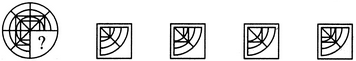(X)                 (1)             (2)              (3)             (4)
Solution: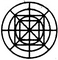QUESTION: 5

In each of the following questions, select a figure from amongst the four alternatives, which when placed in the blank space of figure (X) would complete the pattern.

Question -

Identify the figure that completes the pattern.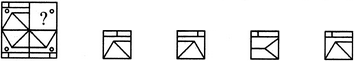(X)                 (1)             (2)              (3)             (4)
Solution: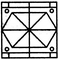QUESTION: 6

In each of the following questions, select a figure from amongst the four alternatives, which when placed in the blank space of figure (X) would complete the pattern.

Question -

Identify the figure that completes the pattern.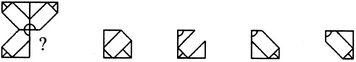(X)                 (1)             (2)              (3)             (4)
Solution: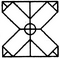QUESTION: 7

In each of the following questions, select a figure from amongst the four alternatives, which when placed in the blank space of figure (X) would complete the pattern.

Question -

Identify the figure that completes the pattern.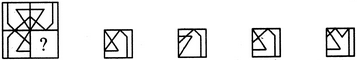(X)                 (1)             (2)              (3)             (4)
Solution: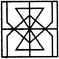QUESTION: 8

In each of the following questions, select a figure from amongst the four alternatives, which when placed in the blank space of figure (X) would complete the pattern.

Question -

Identify the figure that completes the pattern.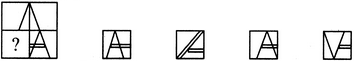(X)                 (1)             (2)              (3)             (4)
Solution: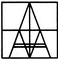QUESTION: 9

In each of the following questions, select a figure from amongst the four alternatives, which when placed in the blank space of figure (X) would complete the pattern.

Question -

Identify the figure that completes the pattern.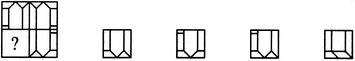(X)                 (1)             (2)              (3)             (4)
Solution: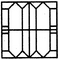QUESTION: 10

In each of the following questions, select a figure from amongst the four alternatives, which when placed in the blank space of figure (X) would complete the pattern.

Question -

Identify the figure that completes the pattern.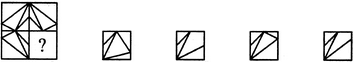(X)                 (1)             (2)              (3)             (4)
Solution: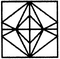QUESTION: 11

In each of the following questions, select a figure from amongst the four alternatives, which when placed in the blank space of figure (X) would complete the pattern.

Question -

Identify the figure that completes the pattern.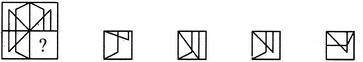(X)                 (1)             (2)              (3)             (4)
Solution: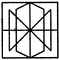QUESTION: 12

In each of the following questions, select a figure from amongst the four alternatives, which when placed in the blank space of figure (X) would complete the pattern.

Question -

Identify the figure that completes the pattern.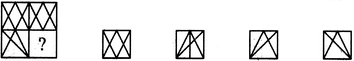(X)                 (1)             (2)              (3)             (4)
Solution: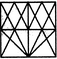QUESTION: 13

In each of the following questions, select a figure from amongst the four alternatives, which when placed in the blank space of figure (X) would complete the pattern.

Question -

Identify the figure that completes the pattern.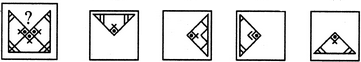(X)                 (1)             (2)              (3)             (4)
Solution: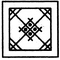QUESTION: 14

In each of the following questions, select a figure from amongst the four alternatives, which when placed in the blank space of figure (X) would complete the pattern.

Question -

Identify the figure that completes the pattern.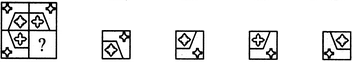(X)                 (1)             (2)              (3)             (4)
Solution: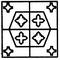QUESTION: 15

In each of the following questions, select a figure from amongst the four alternatives, which when placed in the blank space of figure (X) would complete the pattern.

Question -

Identify the figure that completes the pattern.(X)                 (1)             (2)              (3)             (4)
Solution: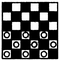QUESTION: 16

In each of the following questions, select a figure from amongst the four alternatives, which when placed in the blank space of figure (X) would complete the pattern.

Question -

Identify the figure that completes the pattern.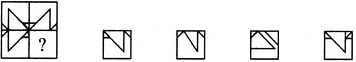(X)                 (1)             (2)              (3)             (4)
Solution: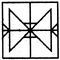QUESTION: 17

In each of the following questions, select a figure from amongst the four alternatives, which when placed in the blank space of figure (X) would complete the pattern.

Question -

Identify the figure that completes the pattern.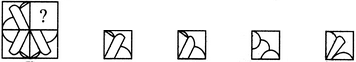(X)                 (1)             (2)              (3)             (4)
Solution: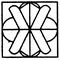QUESTION: 18

In each of the following questions, select a figure from amongst the four alternatives, which when placed in the blank space of figure (X) would complete the pattern.

Question -

Identify the figure that completes the pattern.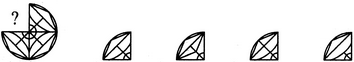(X)                 (1)             (2)              (3)             (4)
Solution: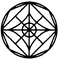QUESTION: 19

In each of the following questions, select a figure from amongst the four alternatives, which when placed in the blank space of figure (X) would complete the pattern.

Question -

Identify the figure that completes the pattern.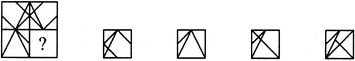(X)                 (1)             (2)              (3)             (4)
Solution: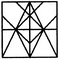QUESTION: 20

In each of the following questions, select a figure from amongst the four alternatives, which when placed in the blank space of figure (X) would complete the pattern.

Question -

Identify the figure that completes the pattern.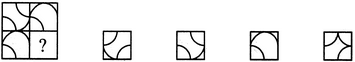(X)                 (1)             (2)              (3)             (4)
Solution: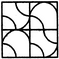QUESTION: 21

In each of the following questions, select a figure from amongst the four alternatives, which when placed in the blank space of figure (X) would complete the pattern.

Question -

Identify the figure that completes the pattern.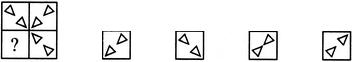(X)                 (1)             (2)              (3)             (4)
Solution: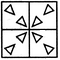QUESTION: 22

In each of the following questions, select a figure from amongst the four alternatives, which when placed in the blank space of figure (X) would complete the pattern.

Question -

Identify the figure that completes the pattern.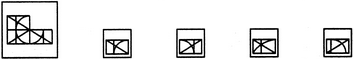(X)                 (1)             (2)              (3)             (4)
Solution: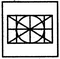QUESTION: 23

In each of the following questions, select a figure from amongst the four alternatives, which when placed in the blank space of figure (X) would complete the pattern.

Question -

Identify the figure that completes the pattern.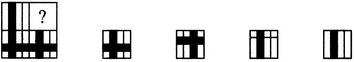(X)                 (1)             (2)              (3)             (4)
Solution: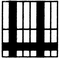QUESTION: 24

In each of the following questions, select a figure from amongst the four alternatives, which when placed in the blank space of figure (X) would complete the pattern.

Question -

Identify the figure that completes the pattern.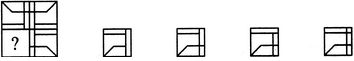(X)                 (1)             (2)              (3)             (4)
Solution: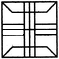QUESTION: 25

In each of the following questions, select a figure from amongst the four alternatives, which when placed in the blank space of figure (X) would complete the pattern.

Question -

Identify the figure that completes the pattern.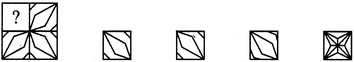(X)                 (1)             (2)              (3)             (4)
Solution: